What are the Number Methods used in ES6 ?

• Last Updated : 18 Oct, 2021

Numbers are one of the primitive data types in JavaScript. JavaScript does not have any specific data type (like int, float, long, etc) for different numbers. It has only one data type i.e. Number. It can be written with or without decimals. They are basically a 64-bit double-precision floating-point value.

There are 6 different Number methods that are used in ES6:

1. Number.isNaN(): It is used to check whether the passed value is NaN or not. If it is a number or string or undefined, it will give false.
Example:

Javascript



Output: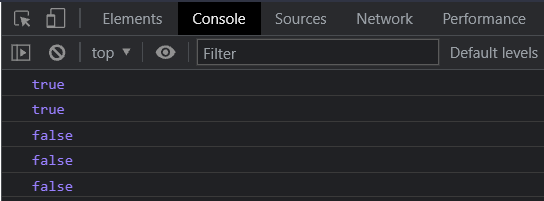Number.isNaN()

2. Number.isInteger(): It is used to determine whether the value passed is a integer or not. The number has to be only integer (negative or positive), else the method will give false.

Example:

Javascript



Output: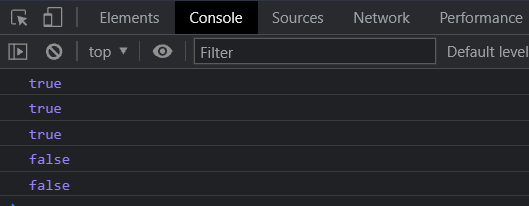Number.isInteger()

3. Number.isSafeInteger(): It Is quite similar to Number.isInteger() in working, as it also determines whether the value passed is integer or not but within the range of -(2^53 – 1) to (2^53 – 1).

Example:

Javascript



Output: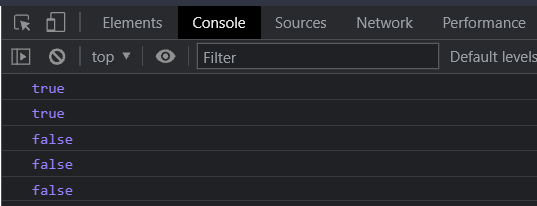Number.isSafeInteger

4. Number.isFinite(): It determines whether the passed value is finite or not. It basically takes a number and check if it is a 64-bit number. If the passed value is a string or null value, it gives. But JavaScript has a global isFinite() also which gives true in all the cases whether it is a number of less than or equal to 64 bits or a string (of a number like “999” and not “Sarthak”) or even a null value.

Example:

Javascript



Output: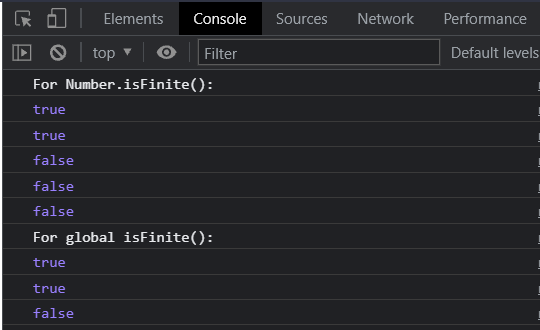Number.isFinite

5. Number.parseFloat() and Number.parseInt(): These two are the most important number methods and they work similarly to their global functions. They parse the passed value to their respective types. parseFloat parses to a float value and parseInt parses to an int value.

Example:

Javascript



Output: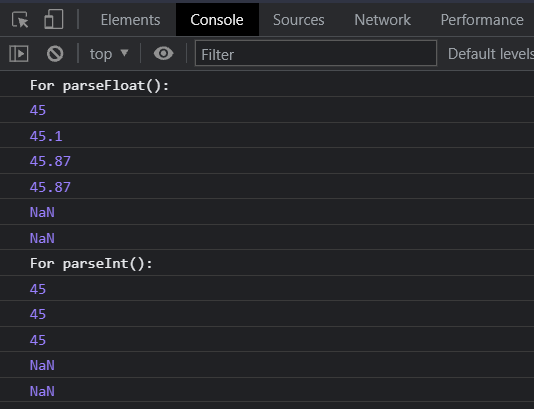parseFloat and parseInt

Hey geek! The constant emerging technologies in the world of web development always keeps the excitement for this subject through the roof. But before you tackle the big projects, we suggest you start by learning the basics. Kickstart your web development journey by learning JS concepts with our JavaScript Course. Now at it's lowest price ever!

My Personal Notes arrow_drop_up